# New Zealand map grid transformation formulae

This page explains how to convert NZMG projection coordinates ( N , E ) to their NZGD1949 geographic equivalents and vice versa.

## Projection parameters

 a Semi-major axis of reference ellipsoid f Ellipsoidal flatteningOrigin latitude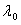Origin longitude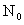False Northing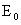False Easting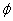Latitude of computation point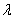Longitude of computation point N Northing of computation point E Easting of computation point

The following equations are divided into three sections:

## NZGD1949 geographic to NZMG projection

The conversion of NZGD1949 geographic coordinates (θ, λto NZMG projection coordinates ( N , E ) is achieved in several steps.

The conversion requires the latitude and longitude expressed in decimal degrees (north and east are positive). The constants are provided on the NZMG page and coefficients used in these formulae are listed below.

Note: NZMG applies to coordinates referenced to NZGD1949. Coordinates from other datums, such as NZGD2000 or WGS84, must be converted to NZGD1949 before these formulae can be applied.

Calculate ΔΨ and Δλ using:

Calculate a complex polynomial function of the complex number θ = ΔΨ + iΔλ using the formula:

Express the complex number z in terms of its real and imaginary parts z = x + iy and calculate the northing N and easting E from x and y as:

## NZMG projection to NZGD1949 geographic

The conversion from NZMG to latitude and longitude involves an iterative approximation to reverse step 2 above. This starts with the easting E and northing N. The steps to calculate latitude and longitude are as follows. Note that the constants and coefficients used in these formulae are listed below. Derive the complex number z as the following steps.

Note: NZMG applies to coordinates referenced to NZGD1949. Coordinates from other datums, such as NZGD2000 or WGS84, must be converted to NZGD1949 before these formulae can be applied.

Determine the complex number θ as a series of approximations θ0 , θ1 , θ2 and so on. The first approximation is:

Successive approximations are obtained by applying the formula:

Two iterations of this formula will give millimetre transformation accuracy.
Express θ in terms of its real and imaginary parts θ = ΔΨ + iΔλ and calculate the latitude and longitude as follows

## NZMG coefficients

Coefficient Real Part Imaginary Part
A1 0.6399175073
A2 -0.1358797613
A3 0.063294409
A4 -0.02526853
A5 0.0117879
A6 -0.0055161
A7 0.0026906
A8 -0.001333
A9 0.00067
A10 -0.00034
B1 0.7557853228 0
B2 0.249204646 0.003371507
B3 -0.001541739 0.041058560
B4 -0.10162907 0.01727609
B5 -0.26623489 -0.36249218
B6 -0.6870983 -1.1651967
C1 1.3231270439 0
C2 -0.577245789 -0.007809598
C3 0.508307513 -0.112208952
C4 -0.15094762 0.18200602
C5 1.01418179 1.64497696
C6 1.9660549 2.5127645
D1 1.5627014243
D2 0.5185406398
D3 -0.03333098
D4 -0.1052906
D5 -0.0368594
D6 0.007317
D7 0.01220
D8 0.00394
D9 -0.0013